# Fraction calculator

The calculator performs basic and advanced operations with fractions, expressions with fractions combined with integers, decimals, and mixed numbers. It also shows detailed step-by-step information about the fraction calculation procedure. Solve problems with two, three, or more fractions and numbers in one expression.

## Result:

### 61/4 + 94/5 = 321/20 = 16 1/20 = 16.05

Spelled result in words is three hundred twenty-one twentieths (or sixteen and one twentieth).

### How do you solve fractions step by step?

1. Conversion a mixed number 6 1/4 to a improper fraction: 6 1/4 = 6 1/4 = 6 · 4 + 1/4 = 24 + 1/4 = 25/4

To find a new numerator:
a) Multiply the whole number 6 by the denominator 4. Whole number 6 equally 6 * 4/4 = 24/4
b) Add the answer from previous step 24 to the numerator 1. New numerator is 24 + 1 = 25
c) Write a previous answer (new numerator 25) over the denominator 4.

Six and one quarter is twenty-five quarters
2. Conversion a mixed number 9 4/5 to a improper fraction: 9 4/5 = 9 4/5 = 9 · 5 + 4/5 = 45 + 4/5 = 49/5

To find a new numerator:
a) Multiply the whole number 9 by the denominator 5. Whole number 9 equally 9 * 5/5 = 45/5
b) Add the answer from previous step 45 to the numerator 4. New numerator is 45 + 4 = 49
c) Write a previous answer (new numerator 49) over the denominator 5.

Nine and four fifths is forty-nine fifths
3. Add: 25/4 + 49/5 = 25 · 5/4 · 5 + 49 · 4/5 · 4 = 125/20 + 196/20 = 125 + 196/20 = 321/20
For adding, subtracting, and comparing fractions, it is suitable to adjust both fractions to a common (equal, identical) denominator. The common denominator you can calculate as the least common multiple of both denominators - LCM(4, 5) = 20. In practice, it is enough to find the common denominator (not necessarily the lowest) by multiplying the denominators: 4 × 5 = 20. In the following intermediate step, the fraction result cannot be further simplified by canceling.
In other words - twenty-five quarters plus forty-nine fifths = three hundred twenty-one twentieths.

#### Rules for expressions with fractions:

Fractions - simply use a forward slash between the numerator and denominator, i.e., for five-hundredths, enter 5/100. If you are using mixed numbers, be sure to leave a single space between the whole and fraction part.
The slash separates the numerator (number above a fraction line) and denominator (number below).

Mixed numerals (mixed fractions or mixed numbers) write as integer separated by one space and fraction i.e., 1 2/3 (having the same sign). An example of a negative mixed fraction: -5 1/2.
Because slash is both signs for fraction line and division, we recommended use colon (:) as the operator of division fractions i.e., 1/2 : 3.

Decimals (decimal numbers) enter with a decimal point . and they are automatically converted to fractions - i.e. 1.45.

The colon : and slash / is the symbol of division. Can be used to divide mixed numbers 1 2/3 : 4 3/8 or can be used for write complex fractions i.e. 1/2 : 1/3.
An asterisk * or × is the symbol for multiplication.
Plus + is addition, minus sign - is subtraction and ()[] is mathematical parentheses.
The exponentiation/power symbol is ^ - for example: (7/8-4/5)^2 = (7/8-4/5)2

#### Examples:

subtracting fractions: 2/3 - 1/2
multiplying fractions: 7/8 * 3/9
dividing Fractions: 1/2 : 3/4
exponentiation of fraction: 3/5^3
fractional exponents: 16 ^ 1/2
adding fractions and mixed numbers: 8/5 + 6 2/7
dividing integer and fraction: 5 ÷ 1/2
complex fractions: 5/8 : 2 2/3
decimal to fraction: 0.625
Fraction to Decimal: 1/4
Fraction to Percent: 1/8 %
comparing fractions: 1/4 2/3
multiplying a fraction by a whole number: 6 * 3/4
square root of a fraction: sqrt(1/16)
reducing or simplifying the fraction (simplification) - dividing the numerator and denominator of a fraction by the same non-zero number - equivalent fraction: 4/22
expression with brackets: 1/3 * (1/2 - 3 3/8)
compound fraction: 3/4 of 5/7
fractions multiple: 2/3 of 3/5
divide to find the quotient: 3/5 ÷ 2/3

The calculator follows well-known rules for order of operations. The most common mnemonics for remembering this order of operations are:
PEMDAS - Parentheses, Exponents, Multiplication, Division, Addition, Subtraction.
BEDMAS - Brackets, Exponents, Division, Multiplication, Addition, Subtraction
BODMAS - Brackets, Of or Order, Division, Multiplication, Addition, Subtraction.
GEMDAS - Grouping Symbols - brackets (){}, Exponents, Multiplication, Division, Addition, Subtraction.
Be careful, always do multiplication and division before addition and subtraction. Some operators (+ and -) and (* and /) has the same priority and then must evaluate from left to right.

## Fractions in word problems:

• ExpressionsLet k represent an unknown number, express the following expressions: 1. The sum of the number n and two 2. The quotient of the numbers n and nine 3. Twice the number n 4. The difference between nine and the number n 5. Nine less than the number n
• How many 3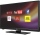How many hours the Andersons watched TV in all Wednesday 3/1 hr Thursdays 2/3 hr Friday 4/5 hr Saturday 3/4 hr
• Cups of flour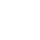Jade was baking cupcakes for her class. She has 4 5/4 cups of flour.  For one batch, she used 1 2/3 cups of flour.  On another batch, she used 7/9 of a cup of flour.  How much flour does Jade have left after making the two batches of cupcakes?
• Ali boughtAli bought 5/6 litre of milk. He drank 1/2 litre and his brother drank 1/6 litre. How much litre of milk left?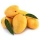A basket contains three types of fruits weighing 87/4 kg in all. If 23/4 kilograms of these are oranges, 48/7 kg are mangoes, and the rest are apples. What is the weight of the apples in the basket?
• Of the 2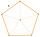Of the shapes,1/6 are triangles and 5/12 are pentagons. What fraction of the shapes are either triangles or pentagons?
• Math homeworkIt took Jose two-thirds of an hour to complete his math homework on Monday, three-fourths of an hour on Tuesday, any two- fifths of an hour on Wednesday. How many hours did it take Jose to complete his homework altogether?
• EvaluateThe division of numbers \$a and \$b increase by-product of the numbers \$c and \$d
• A shopkeeper 3A shopkeeper sells 8 1/3 kg, 10 1/4 kg and 11 1/5 kg of apples on 3 consecutive days. What is the total weight of apples sold?
• A 14.5-gallonA 14.5-gallon gasoline tank is 3/4 full. How many gallons will it take to fill the tank? Write your answer as a mixed number.
• Mac painterMac is mixing red and white paint to make pink for his candy painting. He uses 1/4 ounce of red paint and 2/3 ounce of white paint. How many ounces of paint did he use?Skilled workshop master washes client car 1/5 hour, cleaned the client's car in 5/4 hour, and painted small defects on car 1 1/3 hour. How long did it take him to do all the necessary work tasks?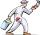Last summer, Mang Tinoy repainted his car. He mixed 2 3/8 cans of white paint, 1 1/3 cans of red paint, and 1 2/4 cans of blue paint. How much paint did he used overall?